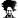Data Table inserting a new blank row and write values

inddon

Member
Hello There,

I have a VBA code which inserts a new row at the end of a data table. It inserts a blank new row at the end of the table but it overwrites the previous row values.

I would like it to write the values to the newly inserted blank row which it created

Please find attached a sample workbook with the code for your reference.

Regards,
Don

Attachments

• 15.3 KB Views: 3

Chihiro

Excel Ninja

Ex:
Code:
``Intersect(O_MstTbl_Transactions.ListColumns("Sr.").DataBodyRange, Maxrr.Offset(1)).Value = MaxSrNumber``

•inddon and Hui

Hui

Excel Ninja
Staff member
Don

Change the last two rows as shown below:

Code:
``````Sub WriteData()
Dim MstWSName_Transactions As Worksheet
Dim MstTblName_Transactions, MaxSrNumber As String
Dim O_MstTbl_Transactions As ListObject

Dim fnd, rngSearch, Maxrr As Range

'Set Master Worksheet and Master Table for Transactions
Set MstWSName_Transactions = ThisWorkbook.Worksheets("Transactions")
MstTblName_Transactions = "Tbl_Transactions"
Set O_MstTbl_Transactions = MstWSName_Transactions.ListObjects(MstTblName_Transactions)

Set Maxrr = O_MstTbl_Transactions.DataBodyRange.Rows(O_MstTbl_Transactions.ListRows.Count)
MaxSrNumber = Intersect(Maxrr.EntireRow, O_MstTbl_Transactions.ListColumns("Sr.").DataBodyRange).Value + 1

'Set Maxrr = O_MstTbl_Transactions.DataBodyRange.Rows(O_MstTbl_Transactions.ListRows.Count + 1).Add

Intersect(O_MstTbl_Transactions.ListColumns("Sr.").DataBodyRange, Maxrr.Offset(1, 0)).Value = MaxSrNumber
Intersect(O_MstTbl_Transactions.ListColumns("Name").DataBodyRange, Maxrr.Offset(1, 0)).Value = "BB"
End Sub``````
The other thing to be careful of is that the Dim line
Dim MstTblName_Transactions, MaxSrNumber As String

Doesn't mean
Dim MstTblName_Transactions As String, MaxSrNumber As String

It actually means
Dim MstTblName_Transactions As Variant, MaxSrNumber As String

Similarily
Dim fnd, rngSearch, Maxrr As Range

means
Dim fnd as variant, rngSearch as variant, Maxrr As Range

•inddon and Chihiro

Chihiro

Excel Ninja
Ah, didn't catch that single line multi variable declaration. Nice catch.

inddon

Member

Ex:
Code:
``Intersect(O_MstTbl_Transactions.ListColumns("Sr.").DataBodyRange, Maxrr.Offset(1)).Value = MaxSrNumber``
Thank you Chihiroinddon

Member
Don

Change the last two rows as shown below:

Code:
``````Sub WriteData()
Dim MstWSName_Transactions As Worksheet
Dim MstTblName_Transactions, MaxSrNumber As String
Dim O_MstTbl_Transactions As ListObject

Dim fnd, rngSearch, Maxrr As Range

'Set Master Worksheet and Master Table for Transactions
Set MstWSName_Transactions = ThisWorkbook.Worksheets("Transactions")
MstTblName_Transactions = "Tbl_Transactions"
Set O_MstTbl_Transactions = MstWSName_Transactions.ListObjects(MstTblName_Transactions)

Set Maxrr = O_MstTbl_Transactions.DataBodyRange.Rows(O_MstTbl_Transactions.ListRows.Count)
MaxSrNumber = Intersect(Maxrr.EntireRow, O_MstTbl_Transactions.ListColumns("Sr.").DataBodyRange).Value + 1

'Set Maxrr = O_MstTbl_Transactions.DataBodyRange.Rows(O_MstTbl_Transactions.ListRows.Count + 1).Add

Intersect(O_MstTbl_Transactions.ListColumns("Sr.").DataBodyRange, Maxrr.Offset(1, 0)).Value = MaxSrNumber
Intersect(O_MstTbl_Transactions.ListColumns("Name").DataBodyRange, Maxrr.Offset(1, 0)).Value = "BB"
End Sub``````
The other thing to be careful of is that the Dim line
Dim MstTblName_Transactions, MaxSrNumber As String

Doesn't mean
Dim MstTblName_Transactions As String, MaxSrNumber As String

It actually means
Dim MstTblName_Transactions As Variant, MaxSrNumber As String

Similarily
Dim fnd, rngSearch, Maxrr As Range

means
Dim fnd as variant, rngSearch as variant, Maxrr As Range
Hi Hui,

Thank you for your solution and also for your recommendations about variable declaration. It is an eye opening. Now I know why there is more memory usgae. I will surely change it in the workbook.Regards,
Don